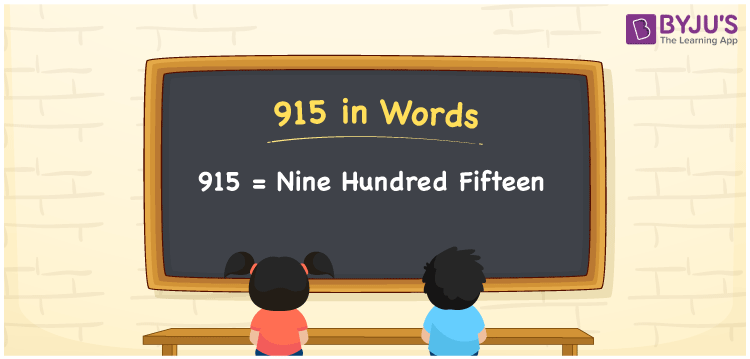# 915 in Words

915 in words is written as Nine Hundred Fifteen. The name of number 915 in English is “Nine Hundred Fifteen”. The word Nine Hundred Fifteen is used in many real-life situations. For example, there are Nine Hundred Fifteen students in a school. Thus, it is a counting number. Also, to represent the currency equal to 915, we can write it in words as Rupees Nine Hundred Fifteen or Nine Hundred Fifteen rupees. Therefore, it is necessary to learn the numbers in words, for the ease of understanding and expressing them.

 915 in words Nine Hundred Fifteen Nine Hundred Fifteen in Numbers 915

## 915 in English Words## How to Write 915 in Words?

If we know the place value of digits of 915, then we can easily express it in words. The place value is basically the position of a digit in a number. 915 is a three-digit number, therefore, we can specify the position of each digit of 915 in a place value chart. In the Indian numbering system, the order of place value of digits from right to left is given by:

 Hundreds Tens Ones 9 1 5

We can write it in expanded form as:

9 × Hundred + 1 × Ten + 5 × One

= 9 × 100 + 1 × 10 + 5 × 1

= 900 + 10 + 5

= 915

= Nine Hundred Fifteen

Therefore, 915 in words is written as eight hundred thirties.

915 is a natural number that precedes 916 and succeeds 914.

915 in words – Nine Hundred Fifteen

Is 915 an odd number? – Yes

Is 915 an even number? – No

Is 915 a perfect square number? – No

Is 915 a perfect cube number? – No

Is 915 a prime number? – No

Is 915 a composite number? – Yes

## Frequently Asked Questions on 915 in Words

### How to write 915 in words?

915 in English is written as “Nine Hundred Fifteen”.

### Is 915 an odd number or even number?

915 is an odd number because it is not wholly divisible by 2.

### Write Nine Hundred Fifteen in numbers.

Nine Hundred Fifteen in numbers is 915.